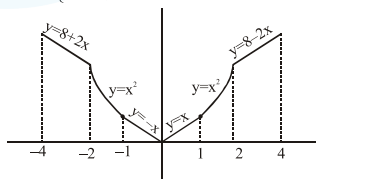# Solve this following`
Question:

Let $f(x)= \begin{cases}\max \left\{|x|, x^{2}\right\}, & |x| \leq 2 \\ 8-2|x|, & 2 Let$S$be the set of points in the interval$(-4,4)$at which$\mathrm{f}$is not differentiable. Then$S$: 1. is an empty set 2. equals$\{-2,-1,1,2\}$3. equals$\{-2,-1,0,1,2\}$4. equals$\{-2,2\}$Correct Option: , 3 Solution:$f(x)= \begin{cases}8+2 x, & -4 \leq x<-2 \\ x^{2}, & -2 \leq x \leq-1 \\ |x|, & -1$f(x)$ is not differentiable at $x=\{-2,-1,0,1,2\}$

$\Rightarrow S=\{-2,-1,0,1,2\}$